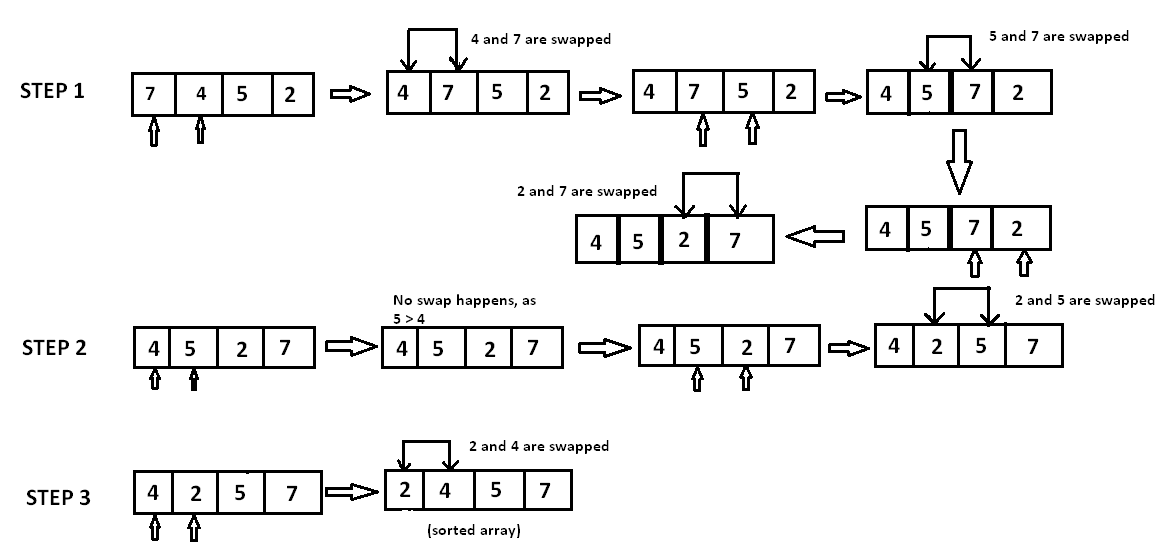Algorithms
Topics:
Bubble Sort
• Searching
• Sorting
• Greedy Algorithms
• Graphs
• String Algorithms
• Dynamic Programming

# Bubble Sort

Sorting Algorithms are concepts that every competitive programmer must know. Sorting algorithms can be used for collections of numbers, strings, characters, or a structure of any of these types.

Bubble sort is based on the idea of repeatedly comparing pairs of adjacent elements and then swapping their positions if they exist in the wrong order.

Assume that $A [ ]$ is an unsorted array of $n$ elements. This array needs to be sorted in ascending order. The pseudo code is as follows:

void bubble_sort( int A[ ], int n ) {
int temp;
for(int k = 0; k< n-1; k++) {
// (n-k-1) is for ignoring comparisons of elements which have already been compared in earlier iterations

for(int i = 0; i < n-k-1; i++) {
if(A[ i ] > A[ i+1] ) {
// here swapping of positions is being done.
temp = A[ i ];
A[ i ] = A[ i+1 ];
A[ i + 1] = temp;
}
}
}
}


Lets try to understand the pseudo code with an example: A [ ] = { 7, 4, 5, 2}In step 1, $7$ is compared with $4$. Since $7 \gt 4$, $7$ is moved ahead of $4$. Since all the other elements are of a lesser value than $7$, $7$ is moved to the end of the array.

Now the array is $A[]=\{4, 5, 2, 7\}$.

In step 2, $4$ is compared with $5$. Since $5 \gt 4$ and both $4$ and $5$ are in ascending order, these elements are not swapped. However, when $5$ is compared with $2$, $5 \gt 2$ and these elements are in descending order. Therefore, $5$ and $2$ are swapped.

Now the array is $A[]=\{4, 2, 5, 7\}$.

In step 3, the element $4$ is compared with $2$. Since $4 \gt 2$ and the elements are in descending order, $4$ and $2$ are swapped.

The sorted array is $A[]=\{2, 4, 5, 7\}$.

Complexity:
The complexity of bubble sort is $O(n^2)$ in both worst and average cases, because the entire array needs to be iterated for every element.

Contributed by: Anand Jaisingh
Уведомления

?